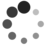# 红黑树 IN Linux （一）

By Chen Jie of TinyLab.org 2016-09-01 21:07:25

## 前言：一杯茶，悠闲看代码## 红黑树：定义

 序号 约束条件 图示 1 红黑树中的节点，非黑即红 2 红黑树根节点为黑色 4 红色节点，其子节点必为黑色 3 所有叶子节点（null）都为黑色 5 由根节点到各叶子节点，路径上的黑色节点一样多

## 红黑树 IN Linux：特点

Linux 表示红黑树的基本结构体为 rb_node，如下图所示：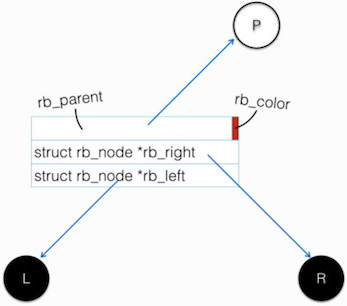Linux 中的红黑树有额外两个特点：

• lockless lookup：即在允许牺牲部分准确性的前提下，无需持有锁，就能进行节点的检索操作。
• augment：augment 是补充的意思，即将每个节点，关联他处的某变量 —— 红黑树发生变化时，通过回调同步调整关联变量。

### lockless lookup

 ```tmp1 = tmp2->rb_left; WRITE_ONCE(sibling->rb_right, tmp1); WRITE_ONCE(tmp2->rb_left, sibling); WRITE_ONCE(parent->rb_left, tmp2);```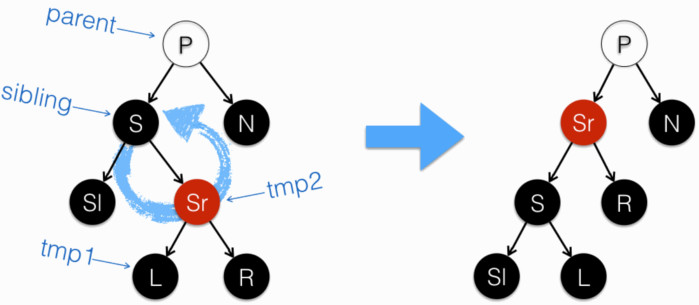1. 把 tmp1（tmp2 的左子）过继给 sibling，做为其右子。tmp2 作为 sibling 原右子，暂时无父节点指向 —— 即从红黑树根节点开始，无法遍历到 tmp2。aka. tmp2 消失
2. tmp2 收 sibling 作为左子，但其自身仍无父节点指向。aka. tmp2 仍然消失，但 tmp2 和 sibling 再次连接
3. parent（原 sibling 的父节点），现指向了 tmp2。aka. tmp2 再次出现

 ```#define WRITE_ONCE(x, val) \ ({ \ union { typeof(x) __val; char __c; } __u = \ { .__val = (__force typeof(x)) (val) }; \ __write_once_size(&(x), __u.__c, sizeof(x));\ __u.__val; \ })``` ```static __always_inline void __write_once_size(volatile void *p, void *res, int size) { switch (size) { case 1: *(volatile __u8 *)p = *(__u8 *)res; break; case 2: *(volatile __u16 *)p = *(__u16 *)res; break; case 4: *(volatile __u32 *)p = *(__u32 *)res; break; case 8: *(volatile __u64 *)p = *(__u64 *)res; break; default: barrier(); __builtin_memcpy((void *)p, (const void *)res, size); barrier(); } }```

• WRITE_ONCE 是在地址 x 上写入值 val。保证“写入”是原子的。
• `__write_once_size` 是实际干活的函数，依据要 x 指向类型的长度，来决定写入方法：
• 此处虽有 `switch(size) ... case `，但 size 在编译时刻就知晓（因为 x 是有类型的），且为内联函数，故实际生成汇编中不存在条件分支。
• 对于基本类型，直接赋值。其原子性由处理器指令，或编译器来保证。
• 注意 `volatile` 在原子操作中出现。
• 对于长度大于 64 字节的类型，“调用” 编译器内建 memcpy 函数。
• 此处虽说是“调用”，其实 gcc 会替换成一组指令来完成拷贝。
• 注意拷贝前后有内存屏障`barrier`，确保 barrier 之后，对内存的更新到达“一致性的 cache 中（即总线上其他人可见）”。
• 再回到 WRITE_ONCE，它没有把 val 直接传给 __write_once_size，而是：
• 构造了一个 union。union 含有一个 char 类型成员 `__c`
• `__write_once_size(&(x), __u.__c, ...`，这么麻烦，没猜错的话是为了 Strict Aliasing

### augment

augment 是一个附加到 rb_node 上的外部变量，当红黑树发生变化（插入/删除节点）时，会通过下述回调，同步 augment：

``````struct rb_augment_callbacks {
void (*propagate)(struct rb_node *node, struct rb_node *stop);
void (*copy)(struct rb_node *old, struct rb_node *new);
void (*rotate)(struct rb_node *old, struct rb_node *new);
};
``````

 ```// http://lxr.free-electrons.com/source/include/linux/rbtree_augmented.h?v=4.7#L57 static inline void rb_insert_augmented(struct rb_node *node, struct rb_root *root, const struct rb_augment_callbacks *augment) { __rb_insert_augmented(node, root, augment->rotate); } // http://lxr.free-electrons.com/source/lib/rbtree.c?v=4.7#L440 void __rb_insert_augmented(struct rb_node *node, struct rb_root *root, void (*augment_rotate)(struct rb_node *old, struct rb_node *new)) { __rb_insert(node, root, augment_rotate); } // http://lxr.free-electrons.com/source/lib/rbtree.c?v=4.7#L418 void rb_insert_color(struct rb_node *node, struct rb_root *root) { __rb_insert(node, root, dummy_rotate); }``` ```// http://lxr.free-electrons.com/source/include/linux/rbtree_augmented.h?v=4.7#L241 static __always_inline void rb_erase_augmented(struct rb_node *node, struct rb_root *root, const struct rb_augment_callbacks *augment) { struct rb_node *rebalance = __rb_erase_augmented(node, root, augment); if (rebalance) __rb_erase_color(rebalance, root, augment->rotate); } // http://lxr.free-electrons.com/source/lib/rbtree.c?v=4.7#L396 void __rb_erase_color(struct rb_node *parent, struct rb_root *root, void (*augment_rotate)(struct rb_node *old, struct rb_node *new)) { ____rb_erase_color(parent, root, augment_rotate); } // http://lxr.free-electrons.com/source/lib/rbtree.c?v=4.7#L424 void rb_erase(struct rb_node *node, struct rb_root *root) { struct rb_node *rebalance; rebalance = __rb_erase_augmented(node, root, &dummy_callbacks); if (rebalance) ____rb_erase_color(rebalance, root, dummy_rotate); }```

``````struct vm_area_struct {
/* The first cache line has the info for VMA tree walking. */

unsigned long vm_start;         /* Our start address within vm_mm. */
unsigned long vm_end;           /* The first byte after our end address
within vm_mm. */

struct vm_area_struct *vm_next, *vm_prev;

struct rb_node vm_rb;

/*
* Largest free memory gap in bytes to the left of this VMA.
* Either between this VMA and vma->vm_prev, or between one of the
* VMAs below us in the VMA rbtree and its ->vm_prev. This helps
* get_unmapped_area find a free area of the right size.
*/
unsigned long rb_subtree_gap;

...
``````

vm_rb 是 vm_area_struct 链入红黑树的“插头”，rb_subtree_gap 就是附加到红黑树节点上的 augment。来看下本例中，rb_augment_callbacks 有哪些？

``````// http://lxr.free-electrons.com/source/mm/mmap.c?v=4.7#L371
RB_DECLARE_CALLBACKS(static, vma_gap_callbacks, struct vm_area_struct, vm_rb,
unsigned long, rb_subtree_gap, vma_compute_subtree_gap)
``````

``````// http://lxr.free-electrons.com/source/include/linux/rbtree_augmented.h?v=4.7#L63
#define RB_DECLARE_CALLBACKS(rbstatic, rbname, rbstruct, rbfield,       \
rbtype, rbaugmented, rbcompute)            \
...                                                                     \
static void                                                             \
rbname ## _rotate(struct rb_node *rb_old, struct rb_node *rb_new)       \
{                                                                       \
rbstruct *old = rb_entry(rb_old, rbstruct, rbfield);            \
rbstruct *new = rb_entry(rb_new, rbstruct, rbfield);            \
new->rbaugmented = old->rbaugmented;                            \
old->rbaugmented = rbcompute(old);                              \
}                                                                       \
rbstatic const struct rb_augment_callbacks rbname = {                   \
rbname ## _propagate, rbname ## _copy, rbname ## _rotate        \
};
``````

``````static long vma_compute_subtree_gap(struct vm_area_struct *vma)
{
unsigned long max, subtree_gap;
max = vma->vm_start;
if (vma->vm_prev)
max -= vma->vm_prev->vm_end;
if (vma->vm_rb.rb_left) {
subtree_gap = rb_entry(vma->vm_rb.rb_left,
struct vm_area_struct, vm_rb)->rb_subtree_gap;
if (subtree_gap > max)
max = subtree_gap;
}
if (vma->vm_rb.rb_right) {
subtree_gap = rb_entry(vma->vm_rb.rb_right,
struct vm_area_struct, vm_rb)->rb_subtree_gap;
if (subtree_gap > max)
max = subtree_gap;
}
return max;
}
``````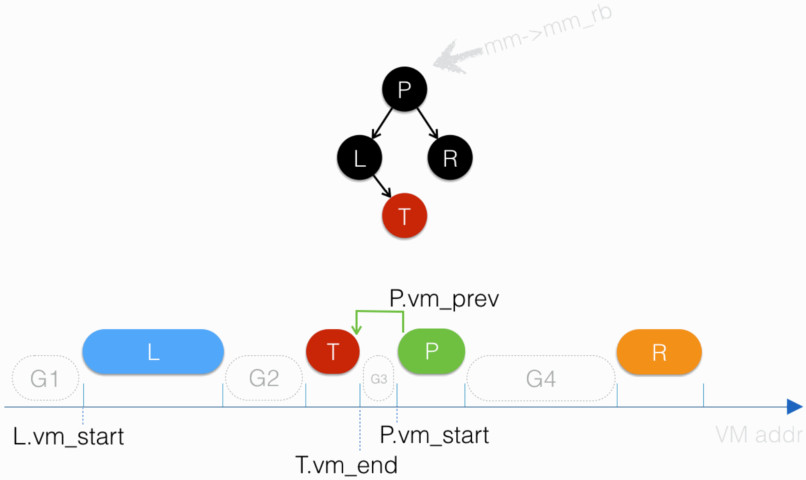• P.vm_start - T.vm_end
• L.rb_subtree_gap
• R.rb_subtree_gap

• T.rb_subtree_gap = T.vm_start - L.vm_end
• R.rb_subtree_gap = R.vm_start - P.vm_end

• 需要分配一个空间（ 图中 斜虚线填充的圆角矩形 ），要求：
• 长度（`vm_unmapped_area_info.length`，对齐到`vm_unmapped_area_info.align_mask`
• 从指定地址区间中分配（`vm_unmapped_area_info.high_limit``vm_unmapped_area_info.low_limit`
• unmapped_area() 试图找到满足要求的、地址最低 的空隙
• 若空隙都太小，且指定地址区间允许，则尝试从最高处（图中 R 之后的空间）分配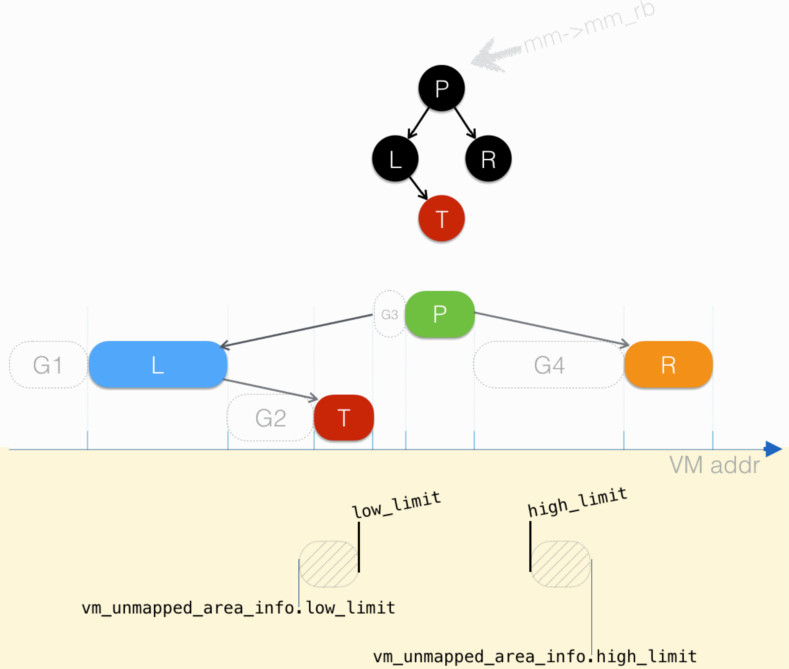``````# 前提假定：待分配的空间，要么可在 P 名下空隙找到；要么没有符合要求的空闲空间
# 伪代码描述：找 P 名下满足分配要求、地址最低的空隙
#
# 出场变量简介：
#   length: 分配请求的长度，对齐到`对齐要求'
#   high_limit: vm_unmapped_area_info.high_limit - length
#   low_limit:  vm_unmapped_area_info.low_limit + length
#
# 要求：
#   gap_start <= high_limit
#   gap_end >= low_limit
#   gap_end - gap_start >= length

# http://lxr.free-electrons.com/source/mm/mmap.c?v=4.7#L1621
gap_end = P.vm_start                # i.e. G3.end

gap_end >= low_limit && P.rb_left && P.rb_left.rb_subtree_gap >= length?
# P.rb_left is L
# L.rb_subtree_gap = max(G1.size, G2.size)
### Current is L !
gap_end = L.vm_start        # i.e. G1.end

# L 没有左子节点

# check_current: http://lxr.free-electrons.com/source/mm/mmap.c?v=4.7#L1634
gap_start = 0               # i.e. G1.start
if (gap_start > high_limit) # 检查：gap_start 必小于等于 high_limit

gap_end >= low_limit?       # i.e. G1.end >= low_limit? answer: no

# Try P.L.T
# http://lxr.free-electrons.com/source/mm/mmap.c?v=4.7#L1641
### Current is T !
gap_end = T.vm_start  # i.e. G2.end

# T 没有左子节点

# check_current
gap_start = L.end     # i.e. G2.start
if (gap_start > high_limit)
gap_end >= low_limit? # i.e. G2.end >= low_limit? answer: no

# T 没有右子节点

# 向上返回...直到返回 P 继续
# 对应代码：http://lxr.free-electrons.com/source/mm/mmap.c?v=4.7#L1652

### Current is P !
gap_start = T.vm_end                # i.e. G3.start
gap_end = P.vm_start                # i.e. G3.end
# check_current
if (gap_start > high_limit)
gap_end >= low_limit?               # answer: yes
gap_end - gap_start >= length?      # i.e. G3.size > length? answer: no

# Try P.R
### Current is R !
gap_end = R.vm_start        # i.e. G4.end

# R 没有左子节点

# check_current
gap_start = P.end           # i.e. G4.start
if (gap_start > high_limit)
gap_end >= low_limit?       # answer: yes
gap_end - gap_start >= length? # i.e. G4.size > length? answer: yes

# found !!!
``````